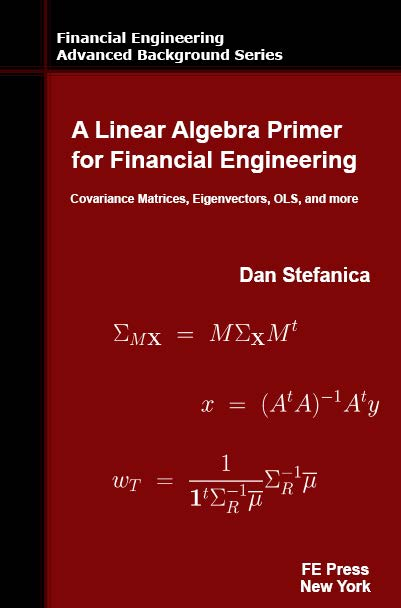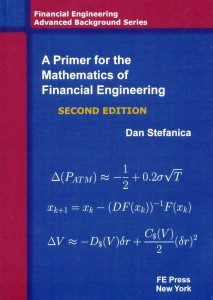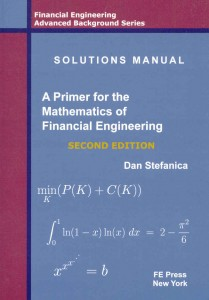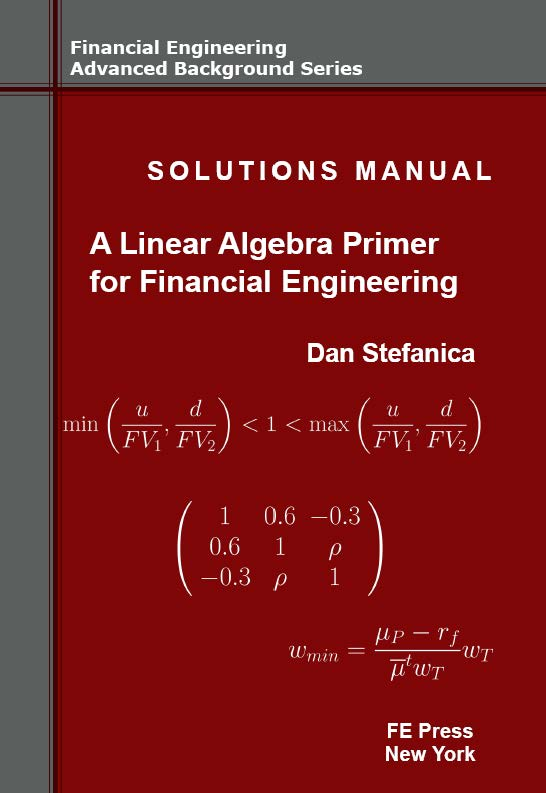# A Linear Algebra Primer for Financial Engineering

#### FE Press offers 15-25% discounts off the list price when two or more books are purchased together. All the books purchased from FE Press are signed and personalized by the authors.

The book “A Linear Algebra Primer for Financial Engineering” is a Top 20 QuantNet bestselling book in 2015 and 2014.A Linear Algebra Primer for Financial Engineering is the third book in the Financial Engineering Advanced Background Series, and covers linear algebra concepts for financial engineering applications from a numerical point of view. The book contains many such applications, as well as pseudocodes, numerical examples, and questions often asked in interviews for quantitative positions.

Sample Sections:

• 7.4. Necessary and sufficient conditions for covariance and correlation matrices (Download)
• 1.1. Column vectors and row vectors. Covariance matrix computation from time series data. (Download)

Book Details: 338 pages; Financial Engineering Press, 2014

Dan Stefanica has been the Director of the Financial Engineering Masters Program at Baruch College, City University of New York, since its inception in 2002, and is the author of the best-selling A Primer For The Mathematics Of Financial Engineering and co-author of 150 Most Frequently Asked Questions on Quant Interviews. He teaches graduate courses on numerical methods for financial engineering, as well as pre-program courses on advanced calculus and numerical linear algebra with financial applications. His research spans numerical analysis, graph theory, and geophysical fluid dynamics.

#### FE Press offers 15-25% discounts off the list price when “A Linear Algebra Primer for Financial Engineering” is purchased together with “A Primer for the Mathematics of Financial Engineering” and its “Solutions Manual”, or with “150 Most Frequently Asked Questions on Quant Interviews”.

All the books purchased from FE Press are signed and personalized by the authors.

Titles Price ORDER• A Primer for the Mathematics of Financial Engineering
• A Linear Algebra Primer for Financial Engineering• A Primer for the Mathematics of Financial Engineering
• A Linear Algebra Primer for Financial Engineering
• 150 Most Frequently Asked Questions on Quant Interviews• A Primer for the Mathematics of Financial Engineering
• Solutions Manual – A Primer for the Mathematics of Financial Engineering• A Linear Algebra Primer for Financial Engineering
• Solutions Manual – A Linear Algebra Primer for Financial Engineering• A Primer for the Mathematics of Financial Engineering
• Solutions Manual – A Primer for the Mathematics of Financial Engineering
• A Linear Algebra Primer for Financial Engineering
• Solutions Manual – A Linear Algebra Primer for Financial Engineering• A Primer for the Mathematics of Financial Engineering
• Solutions Manual – A Primer for the Mathematics of Financial Engineering
• A Linear Algebra Primer for Financial Engineering• A Primer for the Mathematics of Financial Engineering
• A Linear Algebra Primer for Financial Engineering
• Solutions Manual – A Linear Algebra Primer for Financial Engineering• A Primer for the Mathematics of Financial Engineering
• Solutions Manual – A Primer for the Mathematics of Financial Engineering
• 150 Most Frequently Asked Questions on Quant Interviews• A Linear Algebra Primer for Financial Engineering
• Solutions Manual – A Numerical Linear Algebra Primer for Financial Engineering
• 150 Most Frequently Asked Questions on Quant Interviews• A Primer for the Mathematics of Financial Engineering
• 150 Most Frequently Asked Questions on Quant Interviews• A Linear Algebra Primer for Financial Engineering
• 150 Most Frequently Asked Questions on Quant Interviews• A Primer for the Mathematics of Financial Engineering
• Solutions Manual – A Primer for the Mathematics of Financial Engineering
• A Linear Algebra Primer for Financial Engineering
• 150 Most Frequently Asked Questions on Quant Interviews• A Primer for the Mathematics of Financial Engineering
• A Linear Algebra Primer for Financial Engineering
• Solutions Manual – A Numerical Linear Algebra Primer for Financial Engineering
• 150 Most Frequently Asked Questions on Quant Interviews

A Linear Algebra Primer for Financial Engineering is the third book in the Financial Engineering Advanced Background Series, and covers linear algebra concepts for financial engineering applications from a numerical point of view. The book contains many such applications, as well as pseudocodes, numerical examples, and questions often asked in interviews for quantitative positions.List Price: \$62.

Financial Applications

• The Arrow—Debreu one period market model
• One period index options arbitrage
• Covariance and correlation matrix estimation from time series data
• Ordinary least squares for implied volatility computation
• Minimum variance portfolios and maximum return portfolios
• Value at Risk and portfolio VaR

Linear Algebra Topics

• Direct methods for solving linear system. LU and Cholesky decompositions.
• Optimal solvers for tridiagonal symmetric positive matrices
• The Linear Transformation Property
• Multivariate normal random variables
• Efficient cubic spline interpolation
• Ordinary least squares and linear regression
• Gershgorin’s theorem and diagonal dominance
• Sylvester’s criterion

Dan Stefanica has been the Director of the Financial Engineering Masters Program at Baruch College, City University of New York, since its inception in 2002, and is the author of the best-selling A Primer For The Mathematics Of Financial Engineering and co-author of 150 Most Frequently Asked Questions on Quant Interviews. He teaches graduate courses on numerical methods for financial engineering, as well as pre-program courses on advanced calculus and numerical linear algebra with financial applications. His research spans numerical analysis, graph theory, and geophysical fluid dynamics. He taught previously at the Massachusetts Institute of Technology and at New York University. Dan was a silver medalist at the International Mathematics Olympiad, and coached the MIT and NYU teams for the William Lowell Putnam competition.

Sample Sections:

• 7.4. Necessary and sufficient conditions for covariance and correlation matrices (Download)
• 1.1. Column vectors and row vectors. Covariance matrix computation from time series data. (Download)

Data Files:

data-DJ30-july2011-june2013.xlsx

indeces-jul26-aug9-2012.xlsx

indices-july2011.xlsx

spx_may22-jan2015.xlsx

financials2012.xlsx

Book Details:

• Softcover: 338 pages
• Publisher: Financial Engineering Press
• List Price: \$62
• ISBN-13: 978-0979757655
• ISBN-10: 0979757657

Titles from FE Press:A Linear Algebra Primer for Financial Engineering, by Dan Stefanica, FE Press 2014. This book covers linear algebra concepts for financial engineering applications from a numerical point of view. The book contains many such applications, as well as pseudocodes, numerical examples, and questions often asked in interviews for quantitative positions. Table of Contents. This is the third book in the Financial Engineering Advanced Background Series.Solutions Manual – A Linear Algebra Primer for Financial Engineering, by Dan Stefanica, FE Press 2016. This book contains detailed solutions to every one of the 145 exercises from the NLA Primer; Solutions – Chapter 1. This is the fourth book in the Financial Engineering Advanced Background Series.150 Most Frequently Asked Questions on Quant Interviews, by Dan Stefanica, Rados Radoicic, and Tai-Ho Wang. FE Press, 2013. This book contains over 150 questions that are frequently, and also currently, asked on interviews for quantitative positions, covering a vast spectrum, from C++ and data structures, to finance, stochastic calculus and brainteasers. A ten questions selection, with solutions, can be downloaded here. This is the first book in the Pocket Book Guides for Quant Interviews Series.“A Primer for the Mathematics of Financial Engineering”, Second Edition, by Dan Stefanica. FE Press, 2011. This book builds the solid mathematical foundation required to understand the quantitative models used financial engineering and can be used as a reference book or as a self-study book. It contains 175 exercises, many of these being frequently asked interview questions. A Solutions Manual including detailed solutions to every exercise was published concurrently. The Second Edition of A Primer for the Mathematics of Financial Engineering was the Number 1 QuantNet bestselling book of 2013. This is the first book in the Financial Engineering Advanced Background Series.Solutions Manual – A Primer for the Mathematics of Financial Engineering, Second Edition, by Dan Stefanica. FE Press, 2011. 2012. Top 3 QuantNet bestselling book in 2010, 2011, 2012, and 2013. This is the second book in the Financial Engineering Advanced Background Series.